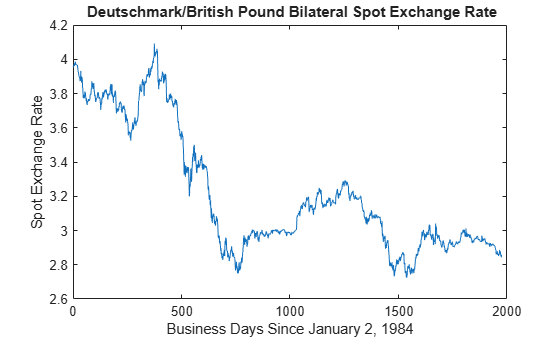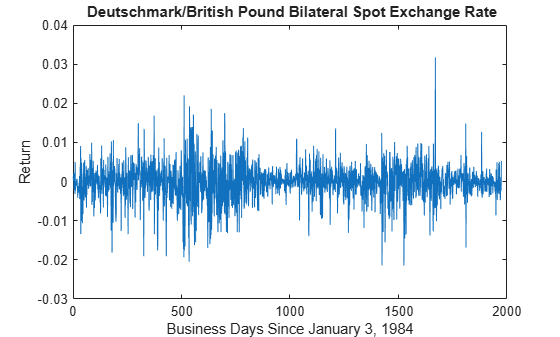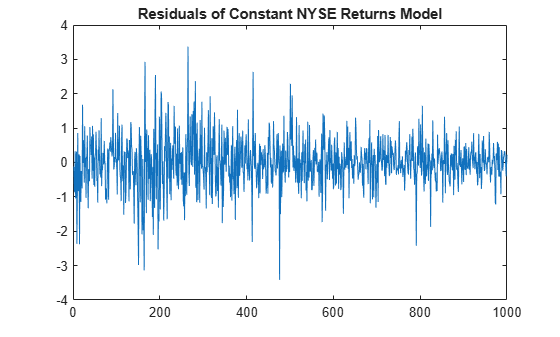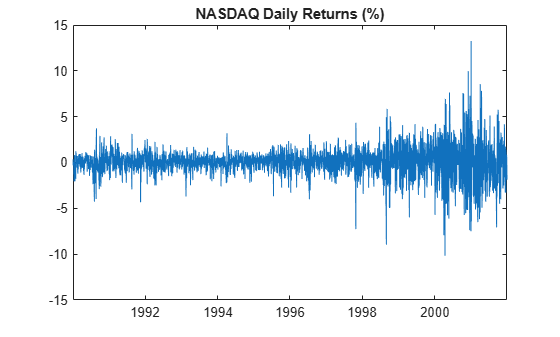# archtest

Engle test for residual heteroscedasticity

## Syntax

h = archtest(res)
[h,pValue,stat,cValue] = archtest(res)
StatTbl = archtest(Tbl)
[___] = archtest(___,Name=Value)

## Description

example

h = archtest(res) returns the rejection decision from conducting Engle’s ARCH test for residual heteroscedasticity in the univariate residual series res.

example

[h,pValue,stat,cValue] = archtest(res) also returns the p-value pValue, test statistic stat, and critical value cValue of the test.

example

StatTbl = archtest(Tbl) returns the table StatTbl containing variables for the test results, statistics, and settings from conducting Engle's ARCH test for residual heteroscedasticity in the last variable of the input table or timetable Tbl. To select a different variable in Tbl to test, use the DataVariable name-value argument.

example

[___] = archtest(___,Name=Value) uses additional options specified by one or more name-value arguments, using any input-argument combination in the previous syntaxes. archtest returns the output-argument combination for the corresponding input arguments. Some options control the number of tests to conduct. The following conditions apply when archtest conducts multiple tests: archtest treats each test as separate from all other tests.If you specify res, all outputs are vectors.If you specify Tbl, each row of StatTbl contains the results of the corresponding test. For example, archtest(Tbl,DataVariable="ResidualGDP",Alpha=0.025,Lags=[1 4]) conducts two tests, at a level of significance of 0.025, for the presence of heteroscedasticity in the variable ResidualGDP of the table Tbl. The first test includes 1 lag in the AR model of the squared residuals, and the second test includes 4 lags.

## Examples

collapse all

Test a time series for ARCH effects using default options of archtest. Input the time series data as a numeric vector.

Load the Deutschmark/British pound foreign-exchange rate data set.

load Data_MarkPound

Data is a time series vector of daily Deutschmark/British pound bilateral spot exchange rates.

Plot the series.

plot(Data) title("\bf Deutschmark/British Pound Bilateral Spot Exchange Rate") ylabel("Spot Exchange Rate") xlabel("Business Days Since January 2, 1984")The series appears nonstationary.

To stabilize the series, convert the spot exchange rates to returns.

returns = price2ret(Data); plot(returns) title("\bf Deutschmark/British Pound Bilateral Spot Exchange Rate") ylabel("Return") xlabel("Business Days Since January 3, 1984")Compute the deviations of the return series from the mean.

residuals = returns - mean(returns);

At 0.05 level of significance, test the residual series of the returns for lag 1 ARCH effects.

h = archtest(residuals)
h = logical 1 

The result h = 1 indicates rejection of the null hypothesis of no conditional heteroscedasticity in favor of a significant lag 1 ARCH effect in the return series.

Load the Deutschmark/British pound foreign-exchange rate data set.

load Data_MarkPound

Preprocess the data by following this procedure:

1. Stabilize the series by computing daily returns.

2. Compute the deviations from the mean return.

returns = price2ret(Data); residuals = returns - mean(returns);

Test the residual series for a significant lag 1 ARCH effect. Return the test decision, $\mathit{p}$-value, test statistic, and critical value.

[h,pValue,stat,cValue] = archtest(residuals)
h = logical 1 
pValue = 0 
stat = 96.2379 
cValue = 3.8415 

Test a time series, which is one variable in a table, for ARCH effects using default options of archtest.

Load the equity index data set Data_EquityIdx. Preprocess the daily NASDAQ closing prices by performing the following actions:

1. Convert the price series to a percentage return series by using price2ret.

2. Represent the series as residuals that fluctuate around a constant level by centering the returns series.

Store the residual series in the table with the rest of the data. Because the price-to-return conversion reduces the sample size from the head of the series, impute the missing residual with the first residual.

load Data_EquityIdx ret = 100*price2ret(DataTable.NASDAQ); res = ret - mean(ret); DataTable.Residuals_NASDAQ = [res(1); res]; DataTable.Properties.VariableNames{end}
ans = 'Residuals_NASDAQ' 

The residual series is the last variable in the table.

Conduct the Engle's ARCH test on the residuals series at a 5% significance level by supplying the entire data set archtest.

StatTbl = archtest(DataTable)
StatTbl=1×6 table h pValue stat cValue Lags Alpha _____ ______ _____ ______ ____ _____ Test 1 true 0 208.1 3.8415 1 0.05 

archtest returns test results and settings in the table StatTbl, where variables correspond to test results (h, pValue, stat, and cValue) and settings (Lags and Alpha), and rows correspond to individual tests (in this case, archtest conducts one test).

h = 1 and pValue = 0 rejects the null hypothesis and suggests that the evidence for ARCH(1) conditional heteroscedasticity in the NASDAQ returns residual series is strong.

By default, archtest tests the last variable in the table. To select a variable from an input table to test, set the DataVariable option.

Conduct several, separate ARCH tests that use different significant levels. Consider the first 1000 days of the daily NYSE closing prices in the equity index data set from Conduct Engle's ARCH Test on Table Variable. Test a time series, which is one variable in a table, for ARCH effects using default options of archtest.

Load the time series data and consider the first 1000 observations. Preprocess and compute the residuals of the NYSE series from a constant only model.

load Data_EquityIdx T = 1000; DataTable = DataTable(1:T,:); ret = 100*price2ret(DataTable.NYSE); res = ret - mean(ret); DataTable.Residuals_NYSE = [res(1); res];

Plot the residuals of the NYSE percent returns series.

plot(1:T,DataTable.Residuals_NYSE) title("Residuals of Constant NYSE Returns Model")The first half of the series appears to have a larger variance than the latter half, which can indicate the presence of volatility clustering.

Conduct the Engle's ARCH test on the residuals series at a 10%, 5%, 1%, and 0.1% significance levels. Specify the table variable name of the residuals.

StatTbl = archtest(DataTable,Alpha=[0.1 0.05 0.01 0.001],DataVariable="Residuals_NYSE")
StatTbl=4×6 table h pValue stat cValue Lags Alpha _____ _________ ______ ______ ____ _____ Test 1 true 0.0058387 7.5994 2.7055 1 0.1 Test 2 true 0.0058387 7.5994 3.8415 1 0.05 Test 3 true 0.0058387 7.5994 6.6349 1 0.01 Test 4 false 0.0058387 7.5994 10.828 1 0.001 

The output table StatTbl contains a row for each test. The test rejects the null hypothesis for each significance level except 0.1% (pValue is the lowest significance level you can use to reject the null hypothesis).

To draw valid inferences from Engle's ARCH test, determine a suitable number of lags for the model by following this procedure:

1. Fit the model over a range of plausible lags.

2. Compare the information criteria of the fitted models.

3. Choose the number of lags that yields the best fitting model for the ARCH test.

Load the equity index data set Data_EquityIdx. Convert the table of data DataTable to a timetable.

load Data_EquityIdx dates = datetime(dates,"ConvertFrom",'datenum'); TT = table2timetable(DataTable,RowTimes=dates); TT.Dates = [];

TT is a timetable containing the same data variable as DataTable, but observations (rows) are associated with the closing times in dates.

Preprocess the daily NASDAQ closing prices by performing the following actions:

1. Convert the price series to a return series by using price2ret.

2. The sampling rate has a relatively high frequency. Therefore, the daily changes can be small. For numerical stability, scale the data by 100.

Store the percent returns series in the table with the rest of the data. Because the price-to-return conversion reduces the sample size from the head of the series, prepend the series with the first percent return.

ret = 100*price2ret(TT.NASDAQ); TT.Returns_NASDAQ = [ret(1); ret]; TT.Properties.VariableNames{end}
ans = 'Returns_NASDAQ' 

Plot the percent returns series.

figure plot(TT.Time,TT.Returns_NASDAQ) title("NASDAQ Daily Returns (%)")The series appears to fluctuate at a constant level. The last quarter of the residual series seems to have higher variance than the first three quarters. This volatile behavior indicates conditional heteroscedasticity.

Fit ARCH Models Over Grid of Lags

Fit an ARCH($\mathit{k}$) model to the NASDAQ percent returns for each $\mathit{k}=0,...,4$. Store the loglikelihood of each fit.

numLags = 4; logL = zeros(numLags,1); % Preallocation for k = 1:numLags Mdl = garch(0,k); [~,~,logL(k)] = estimate(Mdl,TT.Returns_NASDAQ,Display="off"); end

Determine Suitable Number of Lags for Test

Determine the best fitting model by computing and comparing each AIC. Choose the number of lags for the test that corresponds to the best fitting model.

aic = aicbic(logL,1:numLags); [~,lags] = min(aic)
lags = 4 

The best fitting model, according to AIC, has four ARCH lags.

Conduct ARCH Test

Represent the NASDAQ percent returns as residuals that fluctuate around a constant level by centering the returns. Store the returns in the timetable.

TT.Residuals_NASDAQ = TT.Returns_NASDAQ - mean(TT.Returns_NASDAQ);

Conduct Engle's ARCH test at a 1% significance level on the residual series Residuals_NASDAQ. Specify four lags for the test statistic.

StatsTbl = archtest(TT,DataVariable="Residuals_NASDAQ",Lags=lags,Alpha=0.01)
StatsTbl=1×6 table h pValue stat cValue Lags Alpha _____ ______ ______ ______ ____ _____ Test 1 true 0 460.82 13.277 4 0.01 

h = 1 and pValue = 0 rejects the null hypothesis and suggests that the evidence for ARCH(4) conditional heteroscedasticity in the NASDAQ percent returns residual series is strong.

## Input Arguments

collapse all

Residual series, specified as a numeric vector. Each element of res corresponds to an observation.

Typically, res contains the (standardized) residuals from a model fit to observed time series.

Data Types: double

Time series data, specified as a table or timetable. Each row of Tbl is an observation.

Specify a single residual series (variable) to test by using the DataVariable argument. The selected variable must be numeric.

Note

archtest does not support residual series with missing (NaN-valued) observations.

### Name-Value Arguments

Specify optional pairs of arguments as Name1=Value1,...,NameN=ValueN, where Name is the argument name and Value is the corresponding value. Name-value arguments must appear after other arguments, but the order of the pairs does not matter.

Before R2021a, use commas to separate each name and value, and enclose Name in quotes.

Example: Alpha=0.025,Lags=[1 2] conducts two separate tests at a level of significance of 0.025. The first test includes 1 lag in the AR model of the squared residuals, and the second test includes 2 lags.

Number of lags L to include in the AR model for computing the test statistic, specified as a positive integer less than length(res) – 2 or a vector of such positive integers.

archtest conducts a separate test for each element in Lags.

Example: Lags=[1 4] conducts two tests. The first test includes only the first lag in the AR model of the squared residuals, and the second test includes the first through fourth lags.

Data Types: double

Nominal significance level for the hypothesis test, specified as a numeric scalar in the interval (0,1) or a numeric vector of such values.

archtest conducts a separate test for each value in Alpha.

Example: Alpha=[0.01 0.05] uses a level of significance of 0.01 for the first test, and then uses a level of significance of 0.05 for the second test.

Data Types: double

Variable in Tbl to test, specified a string scalar or character vector containing a variable name in Tbl.Properties.VariableNames, or an integer or logical vector representing the index of a name.

Example: DataVariable="ResidualGDP"

Example: DataVariable=[false true false false] or DataVariable=2 tests the second table variable.

Data Types: double | logical | char | string

Note

• When archtest conducts multiple tests, the function applies all single settings (scalars or character vectors) to each test.

• All vector-valued specifications that control the number of tests must have equal length.

• If you specify the vector res and any value is a row vector, all outputs are row vectors.

## Output Arguments

collapse all

Test rejection decisions, returned as a logical scalar or vector with length equal to the number of tests. archtest returns h when you supply the input res.

• Values of 1 indicate rejection of the no ARCH effects null hypothesis in favor of the alternative.

• Values of 0 indicate failure to reject the no ARCH effects null hypothesis.

Test statistic p-values, returned as a numeric scalar or vector with length equal to the number of tests. archtest returns pValue when you supply the input res.

Test statistics, returned as a numeric scalar or vector with length equal to the number of tests. archtest returns stat when you supply the input res.

Test critical values, determined by Alpha, returned as a numeric scalar or vector with length equal to the number of tests. archtest returns cValue when you supply the input res.

Test summary, returned as a table with variables for the outputs h, pValue, stat, and cValue, and with a row for each test. archtest returns StatTbl when you supply the input Tbl.

StatTbl contains variables for the test settings specified by Lags and Alpha.

collapse all

### Engle’s ARCH Test

Engle’s ARCH test assesses the null hypothesis that a series of residuals (rt) exhibits no conditional heteroscedasticity (ARCH effects), against the alternative that an ARCH(L) model describes the series.

The ARCH(L) model has the following form:

${r}_{t}{}^{2}={\alpha }_{0}+{\alpha }_{1}{r}_{t-1}^{2}+\dots \text{+}{\alpha }_{L}{r}_{t-L}^{2}+{e}_{t},$

where there is at least one αj ≠ 0, j = 0,…,L.

The test statistic is the Lagrange multiplier statistic TR2, where:

• T is the sample size.

• R2 is the coefficient of determination from fitting the ARCH(L) model for a number of lags (L) via regression.

Under the null hypothesis, the asymptotic distribution of the test statistic is chi-square with L degrees of freedom.

## Tips

• To draw valid inferences from the test, determine a suitable number of lags by following this procedure:

1. Fit a sequence of ARCH(L) models by using arima, garch, egarch, or gjr models and its corresponding estimate function. Restrict each model by specifying progressively smaller ARCH lags (i.e., ARCH effects corresponding to increasingly smaller lag polynomial terms).

2. Obtain loglikelihoods from the estimated models.

3. Evaluate the significance of each restriction by using lratiotest. Alternatively, compute information criteria using aicbic and combine them with measures of fit.

• Residuals in an ARCH process are dependent, but not correlated. Therefore, archtest tests for heteroscedasticity without autocorrelation. To test for residual autocorrelation, use lbqtest.

• GARCH(P,Q) processes are locally equivalent to ARCH(P + Q) processes. If archtest(res,Lags=L) shows evidence of conditional heteroscedasticity in residuals from a mean model, consider using a GARCH(P,Q) model with P + Q = L.

 Box, George E. P., Gwilym M. Jenkins, and Gregory C. Reinsel. Time Series Analysis: Forecasting and Control. 3rd ed. Englewood Cliffs, NJ: Prentice Hall, 1994.

 Engle, Robert. F. “Autoregressive Conditional Heteroscedasticity with Estimates of the Variance of United Kingdom Inflation.” Econometrica 50 (July 1982): 987–1007. https://doi.org/10.2307/1912773.